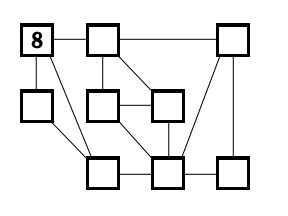All about flooble | fun stuff | Get a free chatterbox | Free JavaScript | Avatarsperplexus dot infoAt Least Three Difference (Posted on 2010-08-04)Place the numbers 1-9 in the boxes so that the difference of each pair of numbers joined by a line is at least three. The number 8 has already been placed.No Solution Yet Submitted by Brian Smith Rating: 5.0000 (1 votes)Comments: ( Back to comment list | You must be logged in to post comments.)solution| Comment 9 of 13 |I know several others have posted already, but here's my thought process used to find a solution. For our purposes, let the empty cells be assigned from left to right, top to bottom with the letters A - H.

Just as Ady did, you must first determine the possible values for cell G. There are two possibilities, 1 or 9. Now, using simple logic, we can see that, if a 9 is there, then five of the numbers 1 - 6 must be linked to that cell. Continuing on, that leaves one value 1 - 6, and a 7 as the values in A and C.

Clearly, 8 - 7 will give us a difference less than the minimum of 3 specified by the problem, and we can conclude that cell G is 1.

With 1 being assigned to cell G, cells B, D, E, F, and H will hold all remaining values > 4. At this point, cells C and F must contain a 2, 3, 4, or 5 in order to be 3 or more away from 8. They must also be at least 3 away from one another, leaving (C, F) = (2, 5) or (C, F) = (5, 2).

F however, must also be at least 3 away from 1 (in cell G), leaving (C, F) = (2, 5). Cell A can now be determined, as it is the only empty cell not linked to cell G, and must take on a value of 3. This leads to an additional finding for cell H, as it must hold the last remaining value less than 3 away from 3, as it is the only vacant cell not linked to cell A. Thus, cell H must hold the number 4.

We have now assigned values as follows:

A = 3, C = 2, F = 5, G = 1, and H = 4. This leaves only 6, 7, and 9 as candidates for cells B, D, and E.

Only one set of values remaining differ by 3 and can occupy cells D and E; 6 and 9. Therefore, cell B holds the 7, and as cells D and E are linked to the same surrounding cells, their values are interchangable.

By following the above steps, we have systematically determined the location of each value in the grid, and all without a single guess.

A = 3, B = 7, C = 2, D = 6/9, E = 9/6, F = 5, G = 1, H = 4

 Posted by Justin on 2010-08-04 23:43:34Please log in:

 Search: Search body:
Forums (0)